Next: Particle in a Box Up: Three-Dimensional Quantum Mechanics Previous: Introduction

# Fundamental Concepts

We have seen that in one dimension the instantaneous state of a single non-relativistic particle is fully specified by a complex wavefunction,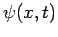. The probability of finding the particle at timebetweenand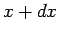is, where(467)

Moreover, the wavefunction is normalized such that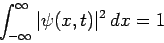(468)

at all times.

In three dimensions, the instantaneous state of a single particle is also fully specified by a complex wavefunction,. By analogy with the one-dimensional case, the probability of finding the particle at timebetweenand, betweenand, and betweenand, is, where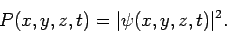(469)

As usual, this interpretation of the wavefunction only makes sense if the wavefunction is normalized such that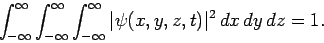(470)

This normalization constraint ensures that the probability of finding the particle anywhere is space is always unity.

In one dimension, we can write the probability conservation equation (see Sect. 4.5)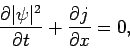(471)

where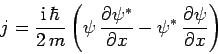(472)

is the flux of probability along the-axis. Integrating Eq. (471) over all space, and making use of the fact that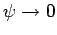asif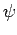is to be square-integrable, we obtain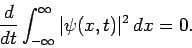(473)

In other words, if the wavefunction is initially normalized then it stays normalized as time progresses. This is a necessary criterion for the viability of our basic interpretation of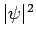as a probability density.

In three dimensions, by analogy with the one dimensional case, the probability conservation equation becomes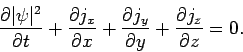(474)

Here,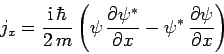(475)

is the flux of probability along the-axis, and(476)

the flux of probability along the-axis, etc. Integrating Eq. (474) over all space, and making use of the fact thatas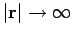ifis to be square-integrable, we obtain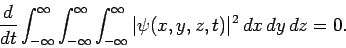(477)

Thus, the normalization of the wavefunction is again preserved as time progresses, as must be the case ifis to be interpreted as a probability density.

In one dimension, position is represented by the algebraic operator, whereas momentum is represented by the differential operator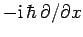(see Sect. 4.6). By analogy, in three dimensions, the Cartesian coordinates,, andare represented by the algebraic operators,, and, respectively, whereas the three Cartesian components of momentum,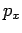,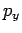, and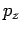, have the following representations: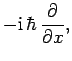(478)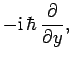(479)(480)

Let,,, and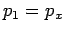, etc. Since the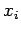are independent variables (i.e.,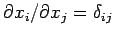), we conclude that the various position and momentum operators satisfy the following commutation relations: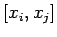(481)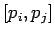(482)(483)

Now, we know, from Sect. 4.10, that two dynamical variables can only be (exactly) measured simultaneously if the operators which represent them in quantum mechanics commute with one another. Thus, it is clear, from the above commutation relations, that the only restriction on measurement in a system consisting of a single particle moving in three dimensions is that it is impossible to simultaneously measure a given position coordinate and the corresponding component of momentum. Note, however, that it is perfectly possible to simultaneously measure two different positions coordinates, or two different components of the momentum. The commutation relations (481)-(483) again illustrate the point that quantum mechanical operators corresponding to different degrees of freedom of a dynamical system (in this case, motion in different directions) tend to commute with one another (see Sect. 6.2).

In one dimension, the time evolution of the wavefunction is given by [see Eq. (199)](484)

where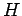is the Hamiltonian. The same equation governs the time evolution of the wavefunction in three dimensions.

Now, in one dimension, the Hamiltonian of a non-relativistic particle of masstakes the form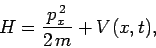(485)

whereis the potential energy. In three dimensions, this expression generalizes to(486)

Hence, making use of Eqs. (478)-(480) and (484), the three-dimensional version of the time-dependent Schröndiger equation becomes [see Eq. (137)]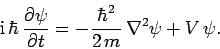(487)

Here, the differential operator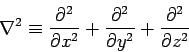(488)

is known as the Laplacian. Incidentally, the probability conservation equation (474) is easily derivable from Eq. (487). An eigenstate of the Hamiltonian corresponding to the eigenvaluesatisfies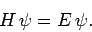(489)

It follows from Eq. (484) that (see Sect. 4.12)(490)

where the stationary wavefunction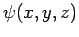satisfies the three-dimensional version of the time-independent Schröndiger equation [see Eq. (295)]: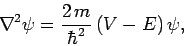(491)

whereis assumed not to depend explicitly on.Next: Particle in a Box Up: Three-Dimensional Quantum Mechanics Previous: Introduction
Richard Fitzpatrick 2010-07-20